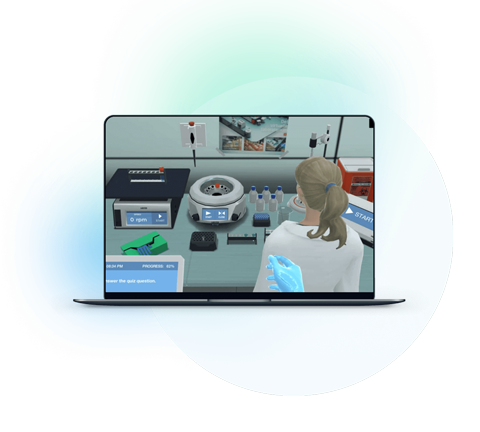Blog

# 5 Physics Lab Techniques Your Students Should Know

Ginelle Testa
Teaching with Labster
October 27, 2022

If you’re teaching a course in physics, perhaps you’re looking for resources to help you introduce or reinforce fundamental physics lab techniques that every student will benefit from.

There are many lab techniques that deal with matter, energy, and their interactions, but we’ve identified six that students would greatly benefit from knowing.

We’ve also identified six Labster virtual labs that help you teach each technique. Not only can your physics students learn the methods, but they can also have fun along the way!

1. Circuit building
2. Pendulum experiment
3. Free-body diagrams
4. Universal Law of Gravitation
5. Quasielastic Neutron Scattering (QENS)

## 6 Physics Techniques

### 1. Circuit building

A circuit is a closed loop that electrons can travel in.  A source of power, such as a battery, provides electrical energy in the circuit. Generally, some appliances use electricity in the circuit that may provide light, heat, or mechanical energy.

#### Labster’s virtual lab that teaches circuit building is:

Electrical Resistance: Apply Ohm's law to simple circuits virtual lab

Students will learn how resistors affect electric current and how modifying the size of a resistor can modify its resistance. They’ll also get the chance to build their own circuit to repair a broken radio to contact their scientist colleagues.

### 2. Pendulum experiment

A simple pendulum assumes the following simplifications:

• The thread has no mass and cannot extend
• The mass attached to the thread is a point mass
• The motion is two-dimensional
• There is no friction or air resistance

#### Labster’s virtual lab that teaches the pendulum experiment is:

Law of Universal Gravitation: Use gravity to orbit the moon virtual lab

To deduce Newton’s Law of Gravitation, students will investigate how gravitational acceleration depends on the masses of objects and the distance between them. Students will learn about the difference between mass and weight. Sir Isaac Newton himself will get on the scales, and you will perform a pendulum experiment that will let you define the gravitational acceleration near the surface of the Earth.

### 3. Free-body diagrams

A free-body diagram is a drawing of all external forces acting on a body. It is a useful tool for analyzing many problems related to forces and motion.

Labster’s virtual lab that teaches the free-body diagrams is:Forces and Free-body Diagrams: Learn how to navigate a drone virtual lab

Students move as a result of forces acting upon them. In this simulation, they’ll learn the basics of common forces as they experience them in everyday life. They’ll visualize the reaction of an object in a given condition using the free-body diagrams.

### 4. Universal Law of Gravitation

This concept is an attractive force that occurs between two masses. There’s a gravitational force between every object in the universe.

#### Labster’s virtual lab that teaches the universal law of gravitation is:

The Gravitational Field: The aliens are coming virtual lab

Students will meet the extraterrestrial Pondus and help him study Earth's gravity. They’ll learn about the gravitational field and acceleration at Earth's surface and anywhere in space and help Pondus understand if Earth is more suitable than his planet for space travel. The mass of his planet is so big that he needs a huge thrust to escape its gravitational pull.

### 5. Quasielastic Neutron Scattering (QENS)

Quasi-elastic neutron scattering (QENS) is a technique used to measure dynamic processes in solid materials such as plastic, ceramics, and gels.

#### Labster’s virtual lab that teaches QENS is:

Students will perform a QENS experiment at a research facility while helping a group of scientists in the Arctic. Observe the structure of atomic layers inside a battery and see how some atoms move when it discharges.

## Wrap-up of techniques

In summary, here are the six physics lab techniques and their accompanying virtual labs:

• How could you incorporate these physics lab techniques into your teaching plans?
• Are these virtual labs better as self-paced homework assignments or small-group collaboration in class?

Interested in these labs? Try them for 30 days with our all-access pass to Labster’s full catalog of virtual labs, including these physics labs!

Reference: